# 针对 GPU 单指令多数据流的编译优化算法GPU

## 1.单指令多数据流

if(A){    B = 1;//Instruction S1    C = 2;//Instruction S2}else{    B = 3;//Instruction S3    C = 4;//Instruction S4}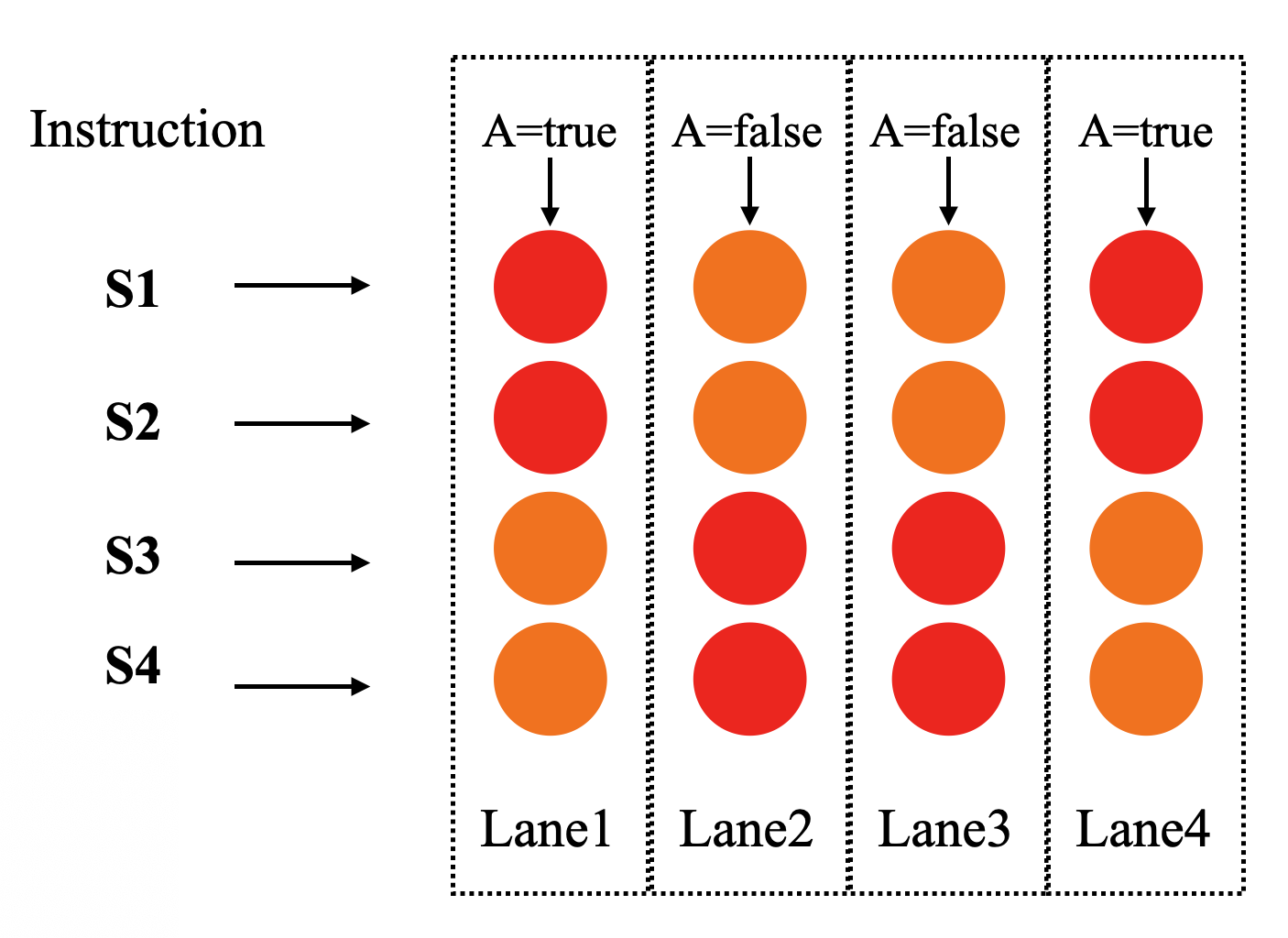GPU单指令多数据流

goto     !A , Labe1;//如果A为false，跳转mov      B , 1;//指令S1mov      C , 2;//指令S2Lable1:mov      B , 3;//指令S3mov      C , 4;//指令S4

(p0) mov      B , 1;//指令S1(p0) mov      C , 2;//指令S2(p1) mov      B , 3;//指令S3(p1) mov      C , 4;//指令S4

## 2.if-conversion算法

1. 计算直接后继支配节点

2. 计算控制依赖CD

3. 计算R&K函数

4. Augment K

R&K分别对应寄存器p的use与def，即寄存器p的使用与定义。

R(x):表示分配给block x的谓词寄存器。block x的执行与否受R(x)中的寄存器控制。也可以说是p的use，即寄存器p用于block x。

K(p):表示谓词寄存器p需要在K(p)中的block中定义。也就是寄存器的def，即寄存器p在那个block定义。

#### 2.1 直接后继支配节点

change = true;//init pdom setpdom(exit_block) = {exit_block}pdom(0:eeit_block-1) = {all blocks}//iterate flow graphwhile(change){  change = false;  for( each block n) with post order  {    tmp = {all blocks};     //求节点n所有直接后继节点的共同后继支配节点    for(each n's successor block p)    {      tmp = tmp & pdom(p);//求交集    }    //n的后继支配节点包括他本身    tmp = tmp | {n};    if(tmp!=pdom(n))    {      pdom(n) = tmp;      change = true;    }  }}

//remove itself from it's pdom setfor each node n{  pdom(n)-={n};}for each node n with post order{  for each s in pdom(n){  //移除直接后继支配节点的后继支配节点     for each t in set( pdom(n)-s ){      if( t is in pdom(s) )        pdom(n)-={t}    }  }}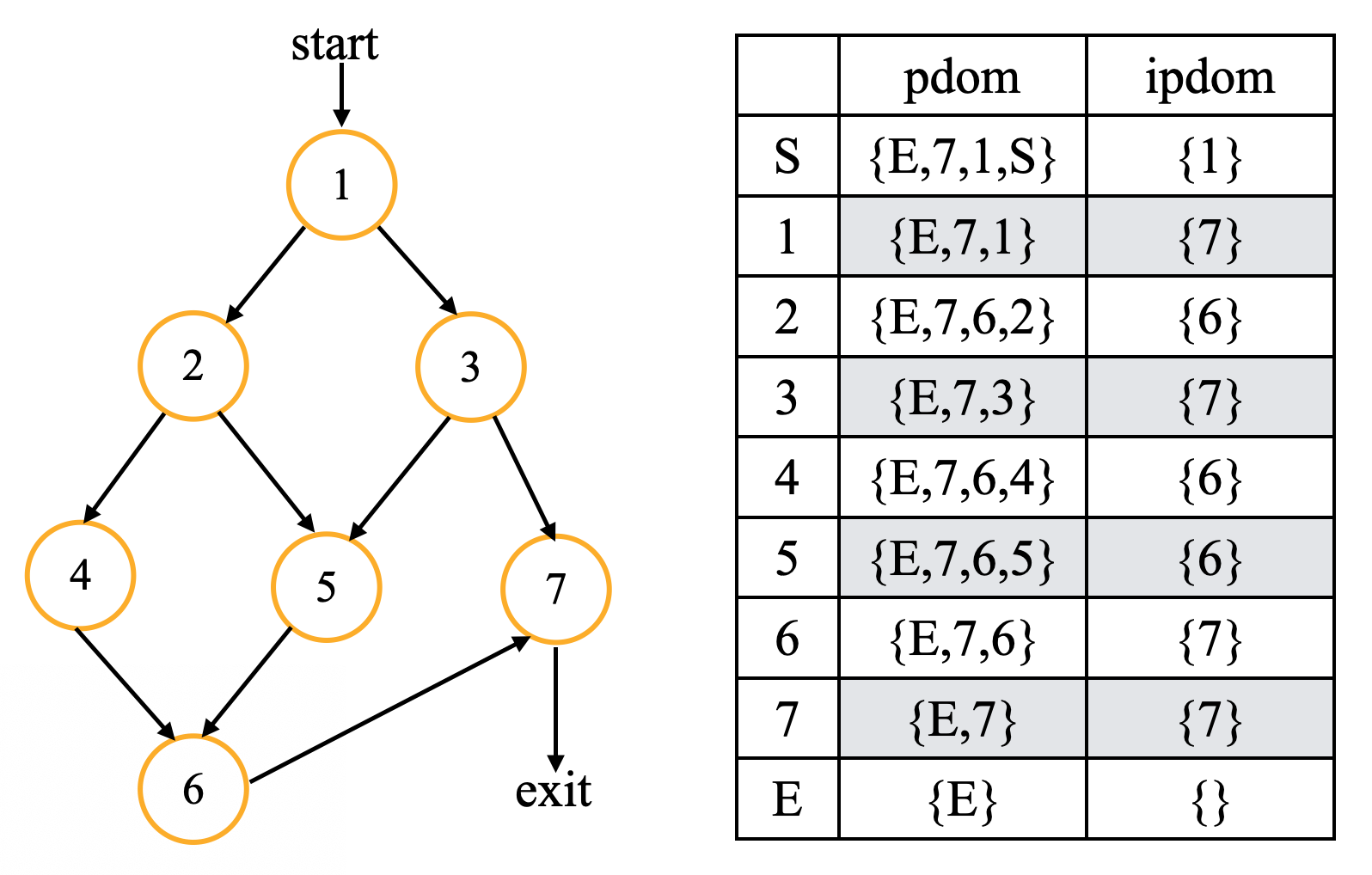#### 2.2. CD

CD是Control Dependent的缩写。直接上英文定义可能更准确一些，详细证明可参考文章末尾给出的论文，公众号后台回复SIMD关键字即可下载。

Y is control dependent on X iff

(1) there exists a directed path P from X to Y with any Z in P (excluding X and Y) post-dominated by Y

(2) X is not post-dominated by Y.

pdom(x) = {y in N: y pdom x}ipdom(x) = {y in N: y ipdom x}for [x,y,label] in E such that y not in pdom(x){    Lub = ipdom(x);    if !label       x = -x    t = y;    while(t!=Lub)    {      CD(t) = CD(t) U {x}//U表示求并集      t = ipdom(t);    }}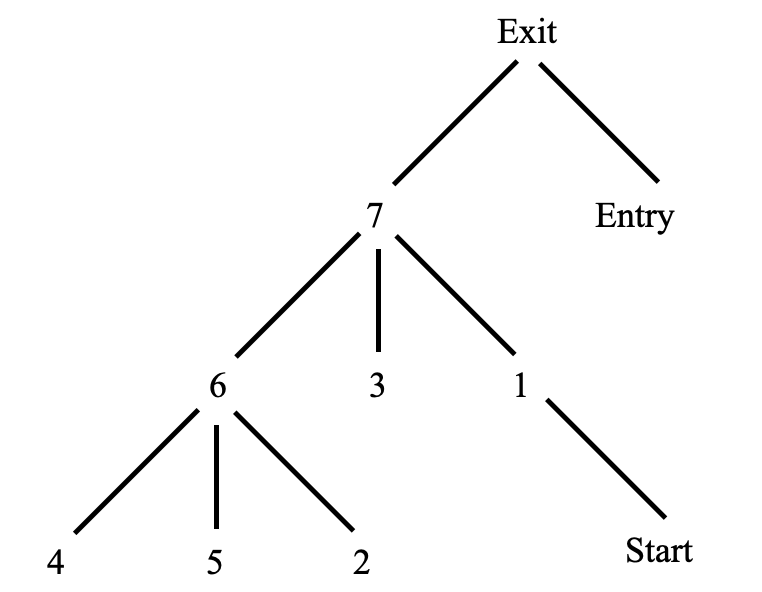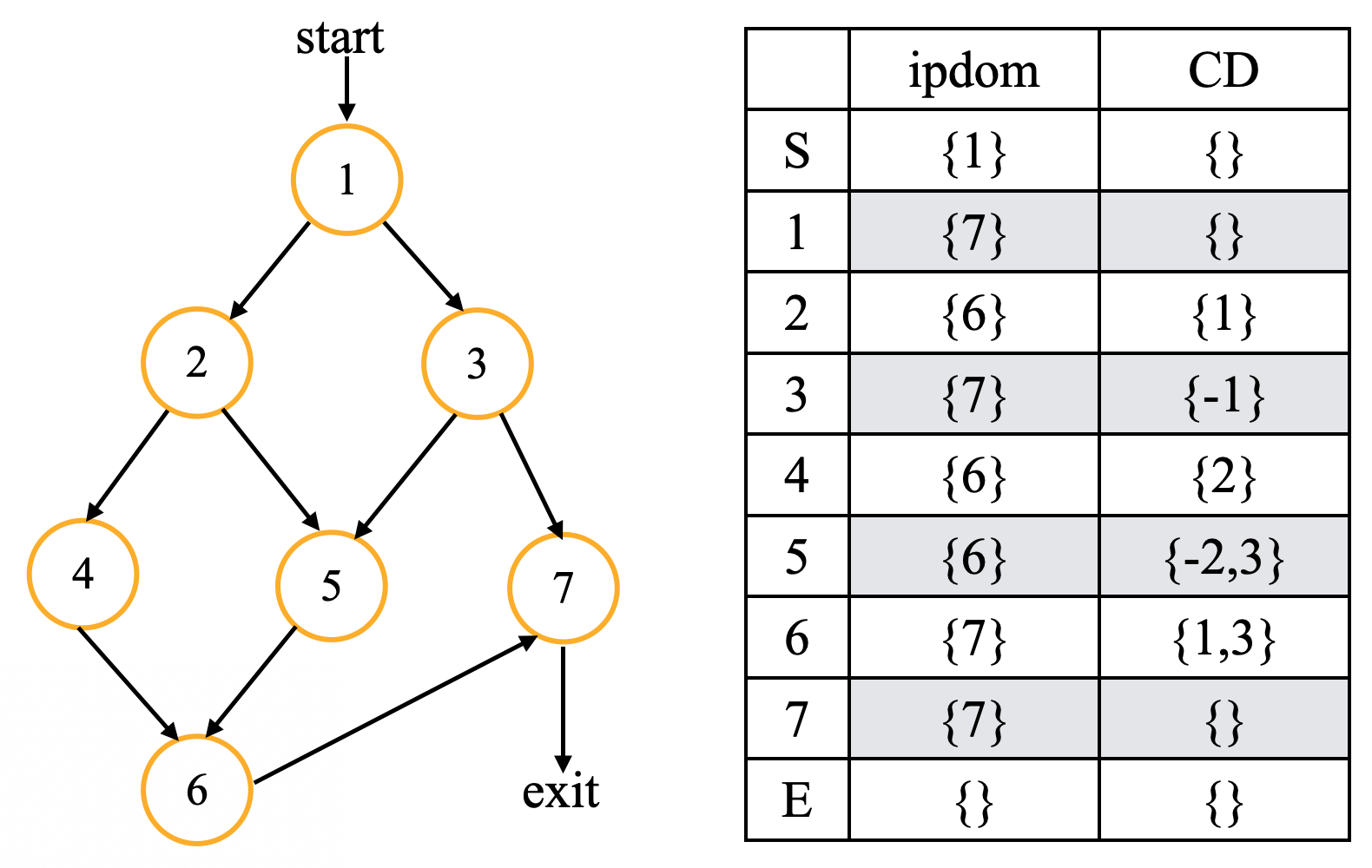CD计算结果

#### 2.3. 计算R&K

p = 1;for x in N    t = CD(x);    if t in K    {        //性质2        R(x) = q such that K(q) = t;    }    else    {        K(p) = t;        R(x) = p++;    }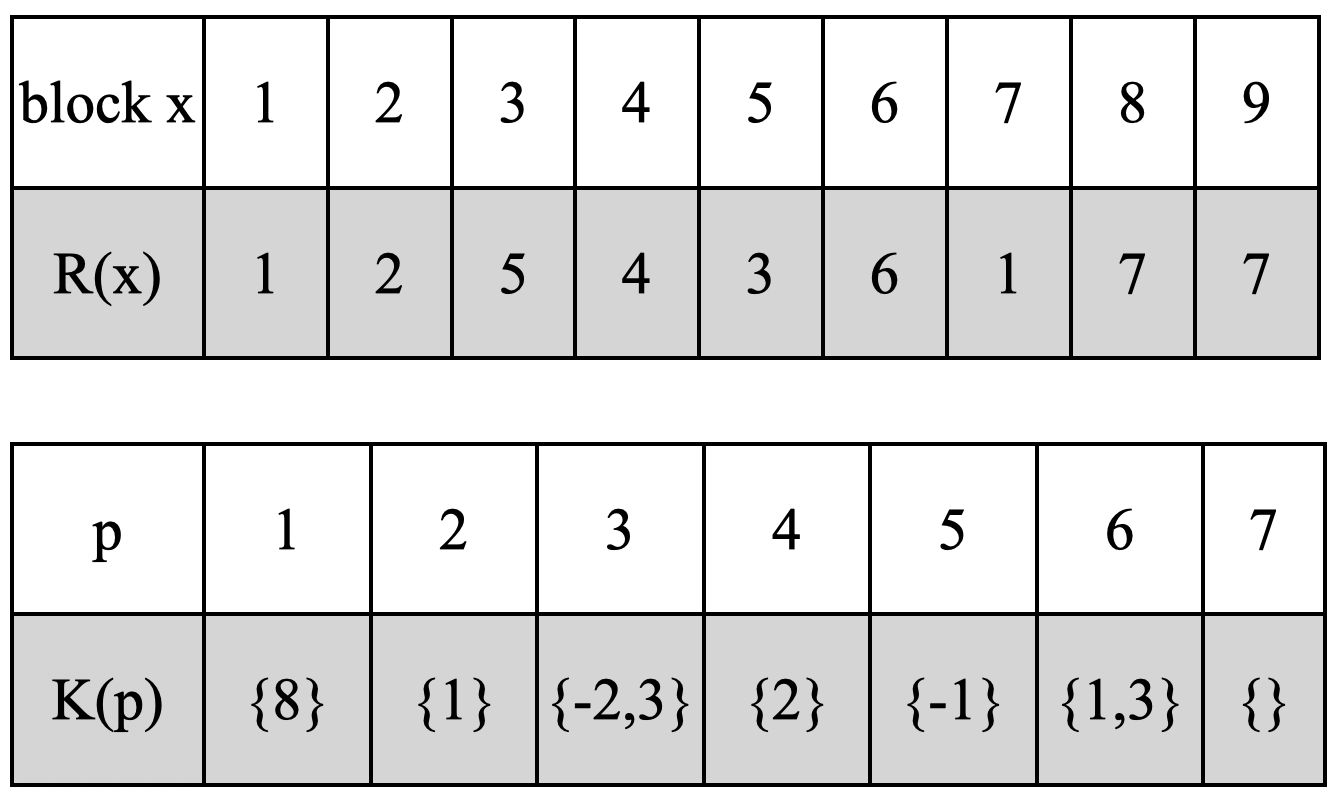R与K的计算结果

#### 2.4. Augment K

k(p)表明p需要在哪些block初始化，但是存在一条路径，刚好没有经过k(p),这个时候p没有被初始化。因此需要在start节点对p进行初始化。

//原始的控制流if(condition1){    block1    if(codition2)    {        block2    }    else    {        block3    }   }

//转换后的顺序执行，是否执行受p寄存器控制（p1) block1;//p2与p3都会在block1中初始化 (p2) block2; (p3) block3;

﻿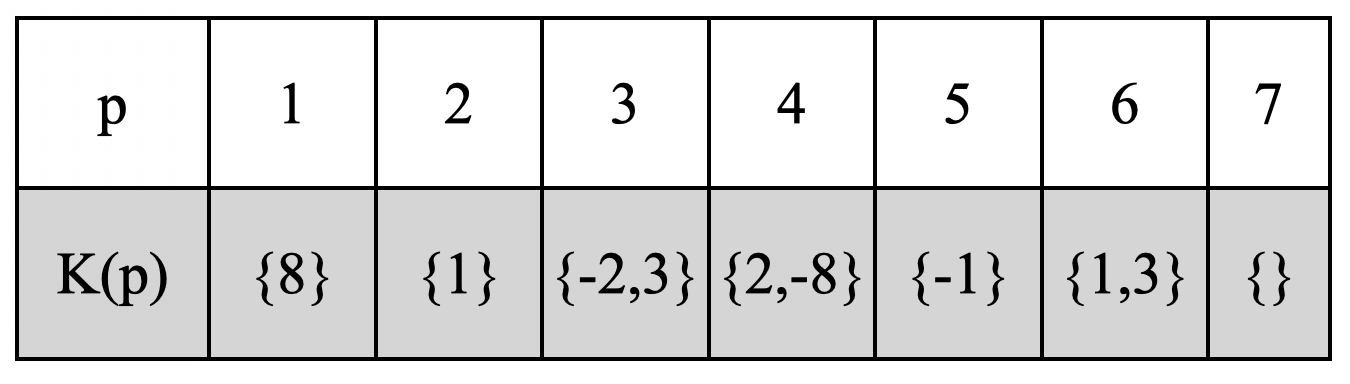Augment K结果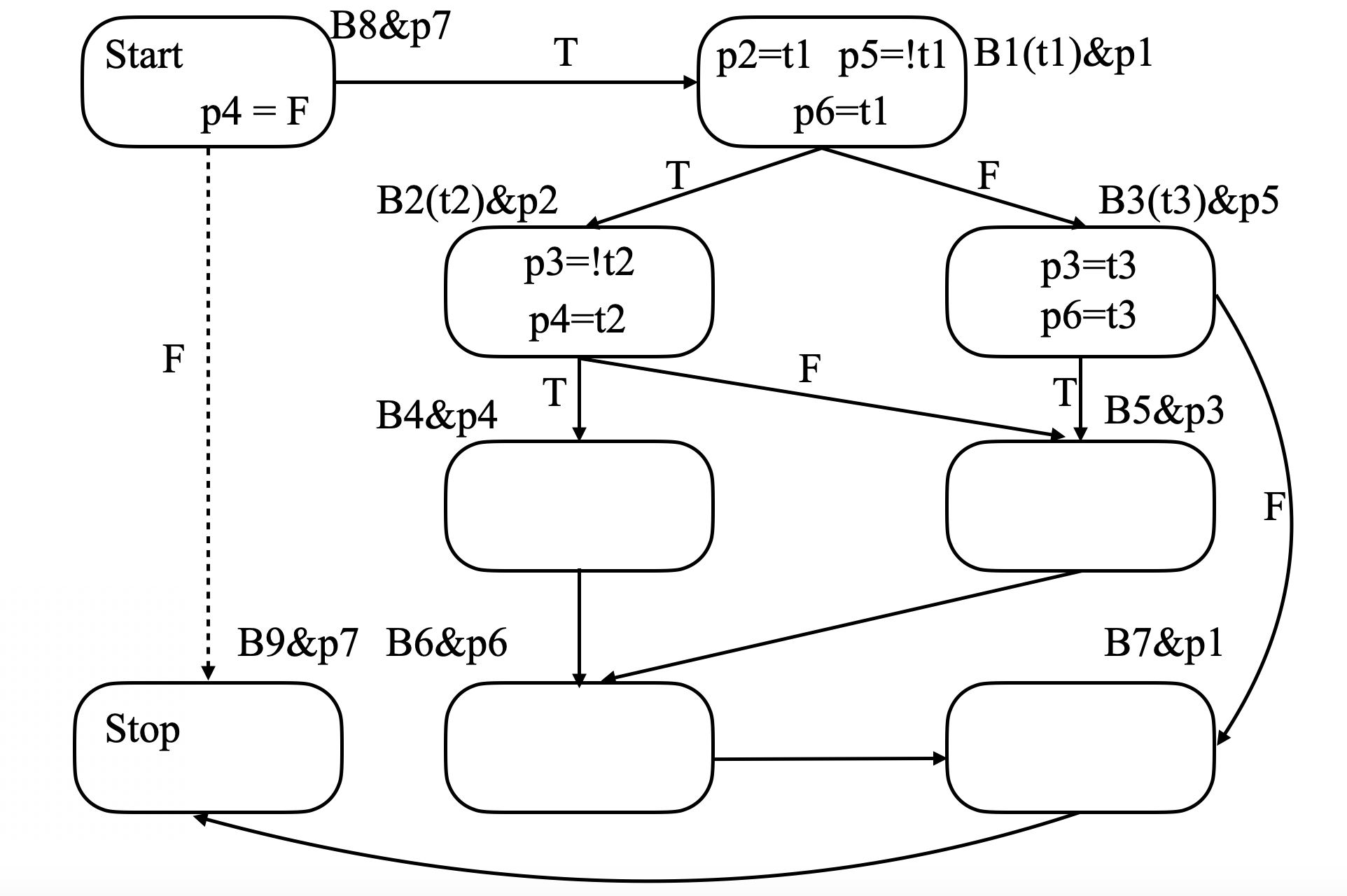p寄存器分配的最后结果

## 3.后记

1.静态单赋值（SSA）

2.基于SSA的稀有条件常数传播（SCCP）

SCCP是后端编译优化中的经典算法。

3.基于图着色的寄存器分配算法

1. 关于支配概念的总结

## 4.往期阅读

﻿

﻿## 评论